# Transition Matrix

## Definition

A Transition Matrix, also, known as a stochastic or probability matrix is a square (n x n) matrix representing the transition probabilities of a stochastic system (e.g. a Markov Chain). The size n of the matrix is linked to the cardinality of the State Space that describes the system being modelled.

### Discrete versus Continuous Time

A fundamental choice of the modelling framework is whether the stochastic system is assumed to evolve in continuous time or in discrete time steps. Continuous time frameworks may be more accurate and/or more mathematically tractable versus discrete time frameworks yet computational representations involve always some form of discrete time approximation.

## Single Step Transitions

In the simplest possible setup, the stochastic system transitions from an initial state to a final state in a single step.

Lets assume a state space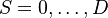$S ={0, \dots ,D}$. If the Transition Probability (the probability of moving from state m to state n) in one time step is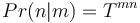$Pr(n|m)=T^{mn}$, the transition matrix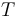$T$ is given by using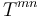$T^{mn}$ as the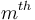$m^{th}$ row and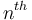$n^{th}$ column element: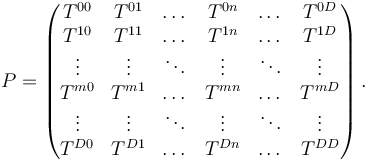$P=\left(\begin{matrix} T^{00} & T^{01} & \dots &T^{0n} & \dots & T^{0D} \\ T^{10} & T^{11} & \dots &T^{1n} & \dots & T^{1D} \\ \vdots & \vdots & \ddots &\vdots & \ddots & \vdots \\ T^{m0} & T^{m1} & \dots &T^{mn} & \dots & T^{mD} \\ \vdots & \vdots & \ddots & \vdots& \ddots & \vdots \\ T^{D0} & T^{D1} & \dots & T^{Dn} & \dots & T^{DD}\\ \end{matrix}\right).$

## Properties

• Since the matrix elements are probabilities they must satisfy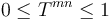$0 \leq T^{mn} \leq 1$
• Since a system must migrate to any of the available states (inclusive of possibly staying in the same state) the transition matrix must satisfy: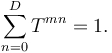$\sum_{n=0}^D T^{mn}=1.\,$

## Characterization

Transition matrices can vary significantly in the type of transition phenomena they capture. Some variations can be captured in well defined mathematical conditions satisfied by the matrix elements

### Markov Chain

In the special case where the system state at a given time is the sole determinant of the probabilities of future transitions we talk about Markov Chains. The Markov assumption is quite strong in general and should be used with care (although its limitations can be sometimes relaxed by extending the state-space)

### Diagonal Dominance

When all diagonal elements of the transition matrix are larger than the corresponding row sum of off-diagonal elements the matrix is called diagonally dominant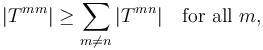$|T^{mm}| \geq \sum_{m\neq n} |T^{mn}| \quad\text{for all } m, \,$

Given that all row elements are non-negative and sum to 1, diagonal dominance is obviously satisfied if the diagonal element is larger than 0.5. Intuitively diagonal dominance implies that the system tends to stay in its current state rather than transition. Diagonally dominant matrices are non-singular, which means that they can be inverted uniquely (this enables among others easy derivation of the transition matrix generator)

### Stochastic Monotonicity

Stochastic Monotonicity is a notion of stochastic ordering. In the context of a transition matrix it expresses the fact that the states are ordered. This means that as a function of the state space index the probability of transitioning to a contiguous sets of states is monotonically increasing: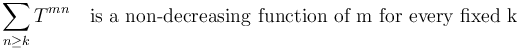$\sum_{n \ge k} T^{mn} \quad\text{is a non-decreasing function of m for every fixed k} \,$

Monotonicity is important when the states aim to express the intrinsic risk of the system (e.g. a credit rating).

### Absorbing States

A state D is called Absorbing Default State if it is impossible to leave this state. Mathematically: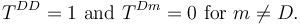$T^{DD} = 1\text{ and }T^{Dm} = 0\text{ for }m \not= D.$

If every state can reach an absorbing state, then the Markov chain is an absorbing Markov chain.

## Multi-Step Transitions

In the general discrete time case, we have a multiplicity of transition matrices, each associated with a given period k: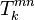$T^{mn}_k$.

### Time Homogeneous Transitions

If the matrix happens to be the same over all periods we talk of time-homogeneous transitions. The assumption of time homogeneity (T is independent of k) would normally not be satisfied. External sector / broader macro-economic factors would make transitions fluctuate.

## Chapman-Kolmogorov Equation

The likelihood of reach a state from an initial time 0 at a final period k is given by the matrix multiplication of the transition matrices of all intermediate steps: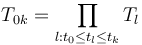$T_{0k} = \prod_{l:t_0 \le t_l \le t_k} T_{l}$

## Generator of a Transition Matrix

The infinitesimal generator Q of a transition matrix T satisfies: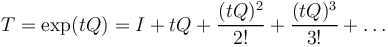$T = \exp(t Q) = I + t Q + \frac{(t Q)^2}{2!} + \frac{(t Q)^3}{3!} + \dots$